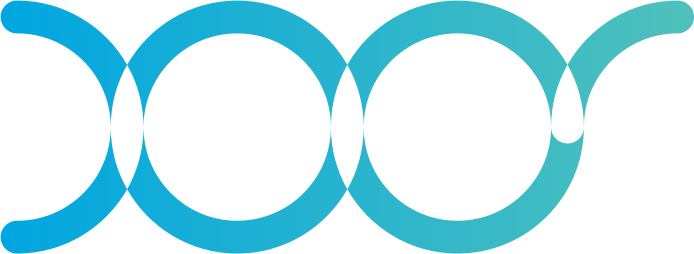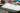# Mastering D3.js (part 2): Basic Zoom and Panning

July 1st, 2018### Building charts that scale with D3.js and Canvas (Part 2)

Written by Maximiliano Duthey, Full Stack Developer @ XOOR

Hi again! Here we are to continue building our scalable plot with D3.js and HTML Canvas. As a super short summary about the previous post, we learned how to draw a scatterplot using D3 on a Canvas instead of the most popular but non-scalable SVG approach.

In the end we got a nice plot but pretty basic and boring as it was fully static, so there was no ability to interact with the plot at all. The goal of this post is to add some interactivity to it by adding panning and zooming functionality.

For this post we’ll continue working on top of the code as we left it in the previous post. Our first step will be to create a function that will allow us to re draw the contents of the canvas with every zoom event.

## The new drawing function

If you remember the last post, we used to draw all points just once whenever the plot was loaded. Since we didn’t have any interactivity, it made sense to do it that way, as the drawing function would be called just one time when the plot was rendered. This is how the code looked like:

 // Draw on canvas dataExample.forEach( point => { drawPoint(point); }); function drawPoint(point) { context.beginPath(); context.fillStyle = pointColor; const px = x(point); const py = y(point); context.arc(px, py, 1.2, 0, 2 * Math.PI,true); context.fill(); }
view raw plot.js hosted with ❤ by GitHub

Now we have a different situation. We want to allow users to do stuff with our plot, so we will need to draw multiple times the points. Why? Well, suppose you drag the plot contents so that it moves and lets you see beyond the initial drew boundaries. Every time such an event occurs, we need to re-draw the canvas contents to make sure we’re responding to the user input. If the user moved the plot 100 pixels to the right side, we need to re-draw everything so that it looks like the plot moved for real.

For this purpose we’ll write a new drawing function that will look like this (don’t panic, we’ll explain what is that transform thing):

 function draw(transform) { const scaleX = transform.rescaleX(x); const scaleY = transform.rescaleY(y); gxAxis.call(xAxis.scale(scaleX)); gyAxis.call(yAxis.scale(scaleY)); context.clearRect(0, 0, width, height); dataExample.forEach( point => { drawPoint(scaleX, scaleY, point, transform.k); }); }
view raw d3-canvas-zoom.js hosted with ❤ by GitHub

As you can see, the forEach loop we had before, is now a bit different. The drawPoint *function now receives 3 extra parameters that we’ll explain soon: *scaleX, scaleY *and transform.k.*

Let’s first explain what our new friend **transform **is: Whenever you execute a panning or a zooming behavior, the visible contents of the canvas will be affected by the new “context” that affects it. This new context is basically how much the plot was moved to each side and how much it was zoomed. All this information resides within the transform object. For more information about transforms, the d3-zoom github page has a quite nice API explanation that might be helpful.

So basically the tranform is an object that beside some methods has 3 main properties:

• transform.x — the translation amount tx along the x-axis. This is, how much the plot should be moved horizontally.
• transform.y — the translation amount ty along the y-axis. This is, how much the plot should be moved vertically.
• transform.k — the scale factor k. This is, how much we should scale the plot when zoom changed. If zoom hasn’t changed, the value of 1 (known as the zoom identity) will be used.

So, now that we understood what a transform is we can continue. The first thing we do in the draw function in lines 2 and 3 is calculating the new scale for the X and Y axis. It’s very important that we don’t override the original scales and store the transformed scales in new variables (more about this here). In order to re-calculate the scales, we use the special functions in the transform object intuitively called rescaleX and rescaleY.

The next step in lines 4 and 6 is to re-draw the X and Y axis applying the new scales that we got. We do that using the *call *function that receives as a parameter the new scale.

Then we clear the canvas, as we will need to re-draw all the points according to the new transformation details. And finally we iterate over our dataset and proceed to draw the points in the canvas.

## The new point drawing function

As we did with everything so far, let’s first look at the final function and then explain the changes made:

 function drawPoint(scaleX, scaleY, point, k) { context.beginPath(); context.fillStyle = pointColor; const px = scaleX(point); const py = scaleY(point); context.arc(px, py, 1.2 * k, 0, 2 * Math.PI, true); context.fill(); }
view raw d3-canvas-zoom-02.js hosted with ❤ by GitHub

As you can see we have those 3 new parameters:

• scaleX, which is the new computed scale for the x axis
• scaleY, which is the new computed scale for the y axis
• k, which is the scale factor that depends on the zoom change

As you can see, now we calculate the values for px and py based on the scaleX and scaleY passed by parameter. This will calculate the new values for the point coordinates that correspond to the current transformation context. Also we calculate a new radius for the point based on the zoom scale factor k, by multiplying the original radius of 1.2 by the scale factor.

Now that we moved the drawing behavior into a function, we need to call the function for the initial drawing, otherwise our plot will be blank. For the initial drawing we will use a special constant that the D3 global object has called **zoomIdentity**. This will draw the plot with no zoom nor X or Y axis transformations. So we’ll place the following call right after the draw function closing bracket.

 draw(d3.zoomIdentity),
view raw d3-canvas-zoom-03.js hosted with ❤ by GitHub

## The zoom and panning handler

We have our new drawing function in place, now it’s time to create some handler that will respond to the user input and will call the improved draw function. Let’s have a look at that handler:

 const zoom_function = d3.zoom().scaleExtent([1, 1000]) .on('zoom', () => { const transform = d3.event.transform; context.save(); draw(transform); context.restore(); }); canvasChart.call(zoom_function);
view raw d3-canvas-zoom-04.js hosted with ❤ by GitHub

We create a variable called *zoom_function *that will be assigned with the zoom behavior so we can reuse it wherever we want, but we just define it once. For the zoom event we use the d3-zoom module which provides the necessary functions to handle zoom and panning events.

So first we call the **d3.zoom() **function, then we chain the **scaleExtent() **call where we set the zooming scale. We set this scale from 1 to 1000, feel free to experiment with whatever values you want here and see how the plot behaves. Finally we define the event handler function which does the following:

1. We get the transformation object
2. We call the draw function passing the transformation

Easy right? :)

Lastly we need to assign the handler to someone, otherwise it’s just a dead variable that nobody will call. In our case, we’re interested on applying zoom and panning to the canvas object, so that’s what we do in the last line by using the call method. This will assign the zoom handler to the chart.

That’s it! You can now open the index.html in your favorite browser and check your shiny new plot with zoom and panning. Guess it’s pretty obvious, but you can zoom with your mouse wheel and pan by click+dragging the plot around.

## Resetting to the initial state

We feel that building this was so easy that we need to add an extra feature to it :)

What we’ll do now, is add a button on the right side of the plot to reset the canvas to the initial state. So that users can play with the zoom and panning features, but also put the plot again back into the initial state without refreshing the page.

The first thing we have to do is adding some self-explaining html to the index file:



Next we’ll add some other self-explaining code to the styles.css file:

 .tools { position: absolute; left: calc(50% + 400px); visibility: hidden; }

We’ll keep our tools box hidden until the script has loaded.

Now we need to implement the javascript code for the reset button functionality. Move to our plot.js file and add the following code:

 const toolsList = container.select('.tools') .style('margin-top', margin.top + 'px') .style('visibility', 'visible'); toolsList.select('#reset').on('click', () => { const t = d3.zoomIdentity.translate(0, 0).scale(1); canvasChart.transition() .duration(200) .ease(d3.easeLinear) .call(zoom_function.transform, t) });
view raw d3-canvas-zoom-06.js hosted with ❤ by GitHub

What we’re doing here is:

1. Grab the tool box container using the select function which we already explained in the previous post.
2. We then apply some margin to it to keep it top aligned with the plot.
3. Finally we reveal the toolbox by setting the visibility to visible.

Now we move inside the toolbox and do the following:

1. We use again the select function but this time we grab our “Reset” button by it’s ID
2. We assign a click handler to our button
3. The click handler first creates the zoom identity transformation to re-draw the plot as we did when drawing it for the first time
4. We then use a transition in the canvas to animate the drawing from the current state to the initial “identity” state. This animation will last 200ms and will use a linear easing function as we usually do with css transitions. You can get more info about transitions with D3 here.
5. Once the transition was defined, we call the zoom handler function by using the transformation defined in step 3.

And we’re done! We now have zoom, panning and an awesome reset button to start all over again :)

## The result

In the end you should get a plot that looks like the one here.

Things started to look more interesting now that we can interact with the plot. There are still a few other things that we’d like to add to the scatterplot like box zooming and some tooltips to allow users to see the values behind each point. But we’ll leave those for the upcoming posts!

Thanks for reading, we hope you’re enjoying and learning as we did with this series and look forward to see your comments! And if you liked it, share it!